P R O F I L EG A L L E R YO R D E RC O N T A C T    I N F OF E A T U R E D   E X H I B I T :    " S T I C K S "

"In the heat of controversy, the forestry and environmental
groups fight to find balance between industry and nature.
Caught in the middle is a way of life, a culture born of
machinery and man."

F E A T U R E D   E X H I B I T : "STICKS
> I M A G E S    1  -  6
>  S E E   I M A G E S    7  -  1 2

>  B A C K   T O   C U R R E N T   F E A T U R E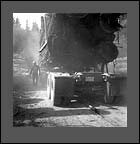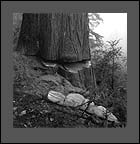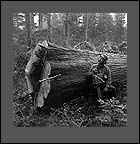d u s tt h e   w e d g e" s t e v e "   f a l l e r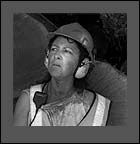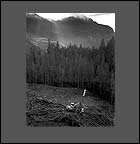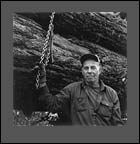" c h a r l e n e "   c h a s e rt h e   s e t t i n g" e d "   d r i v e r
S E E   I M A G E S    7  -  1 2

>  S E N D   T H I S   S I T E   T O   A   F R I E N DT O P© 2001 - 2005   R o b e r  t   J   W i l k i n s o n             P r i v a c y   P o l i c ys i t e   d e s i g n